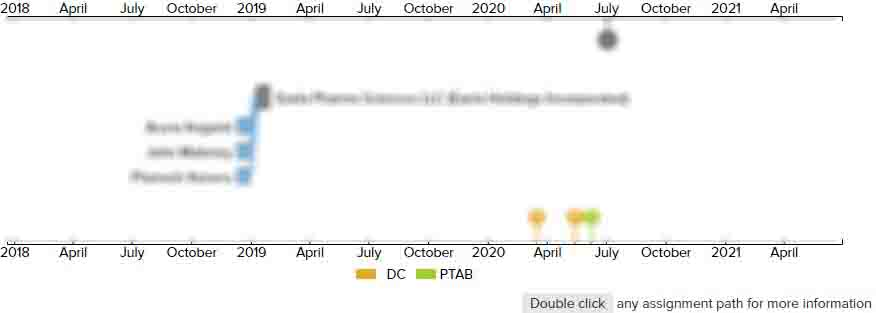Tool path generation method and machine tool using rate of change of curvature based smoothing

• US 10,248,101 B2
• Filed: 10/29/2014
• Issued: 04/02/2019
• Est. Priority Date: 10/29/2014
• Status: Active Grant
First Claim
Patent Images

1. A method of machining a workpiece with a machine tool using a second tool path generated by performing smoothing treatment with respect to a first tool path for machining the workpiece with the machine tool, the method comprising:

• generating the first tool path in which movement points, each associated with a coordinate value, are connected by straight lines;

a step of identifying the movement points in the first tool path;

a rate-of-change calculation step for calculating a rate of change in curvature of the first tool path at each of the movement points;

a weight calculation step for calculating a weight of a moving average at each of the movement points based on the rate of change in the curvature of the first tool path at each of the movement points;

the moving average being based on the respective movement point and a number of adjacent movement points on the first tool path and the weight, and the weight comprising a weight distribution that is adjusted so that smoothing increases as the corresponding rate of change of curvature increases;

a step of calculating a coordinate value of the moving average of each of the movement points by using the weight at each of the movement points and coordinate values of the movement point and the number of adjacent movement points;

setting the coordinate value of the moving average of each movement point as a coordinate value of a corresponding movement point of the second tool path; and

machining the workpiece along the second tool path.

• 1 Assignment
Timeline View
Assignment View×
×